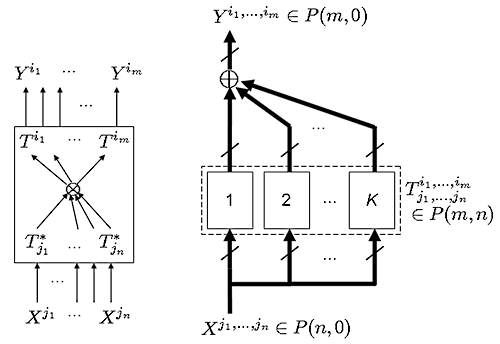### tensors

This has been a confusing topic, with half a dozen Wikipedia pages on the subject. Here I took some notes.

Tensors are sums of “products” of vectors. There are different kinds of vector products. The one used to build tensors is, naturally, the tensor product. In the Cartesian product of vector spaces $$V\times W$$, the set elements are tuples like $$(v,w)$$ where $$v\in V, w\in W$$. A tensor product $$v\otimes w$$ is obtained by tupling the component bases rather than the component elements. If $$V$$ has basis $$\{e_i\}_{i\in\{1,…,M\}}$$ and $$W$$ has basis $$\{f_j\}_{j\in\{1,…,N\}}$$, then take $$\{(e_i,f_j)\}_{i\in\{1,…,M\},j\in\{1,…,N\}}$$ as the basis of the tensor product space $$V\otimes W$$. Then define the tensor product $$v\otimes w$$ as

(1) $$\sum_{i,j} v_i w_j (e_i,f_j) \in V\otimes W$$,

if $$v=\sum_i v_i e_i$$ and $$w=\sum_j w_j f_j$$. The entire tensor product space $$V\otimes W$$ is defined as sums of these tensor products

(2) $$\{\sum_k v_k\otimes w_k | v_k\in V, w_k\in W\}$$.

So tensors in a given basis can be represented as multidimensional arrays.

$$V\otimes W$$ is also a vector space, with $$MN$$ basis dimensions (c.f. $$V\times W$$ with $$M+N$$ basis dimensions). But additionally, it has internal multilinear structure due to the fact that it is made of component vector spaces, namely:

$$(v_1+v_2)\otimes w = v_1\otimes w + v_2\otimes w$$
$$v\otimes (w_1+w_2) = v\otimes w_1 + v\otimes w_2$$
$$\alpha (v\otimes w) = (\alpha v)\otimes w = v\otimes (\alpha w)$$

Higher-order (n-th order) tensor products $$v_1\otimes v_2\otimes \cdots \otimes v_n$$ are obtained by chaining in the obvious way, likewise for higher-order tensor product spaces $$V_1\otimes V_2\otimes \cdots \otimes V_n$$. With this, concatenation of tensors are also defined, i.e. $$S_{i_1,…i_m} \in V_1\otimes \cdots \otimes V_m$$ and $$T_{i_{m+1},…,i_n} \in V_{m+1}\otimes \cdots \otimes V_n$$, then $$S_{i_1,…,i_m}\otimes T_{i_{m+1},…,i_n} = Z_{i_1,…,i_n} \in V_1\otimes \cdots \otimes V_n$$. In other words, the indices are appended. This is essentially the Kronecker product, which generalizes the outer product.

However, usually when tensors are mentioned, the tensor product spaces under discussion are already specialized to those generated from a single base vector space $$V$$ and its dual space $$V^*$$, rather than from a collection of arbitrary vector spaces. In such a space $$P(m,n) = \overbrace{V\otimes \cdots \otimes V}^{m} \otimes \overbrace{V^*\otimes \cdots \otimes V^*}^{n}$$, the component spaces (and their bases, indices, etc.) naturally belong to two groups, those from $$V$$ are called contravariant, those from $$V^*$$ are called covariant, and an (m,n)-tensor from $$P(m,n)$$ is written $$T^{i_1,…,i_m}_{j_1,…,j_n}$$.

This specialization allows the contraction of tensors to be defined. A contraction basically chooses one covariant vector component and one contravariant vector component from a tensor and applies the former as a functional on the latter, e.g., contracting $$T^{i_1,…,i_m}_{j_1,…,j_n} = v_{i_1}\otimes \cdots \otimes v_{i_m} \otimes v^*_{j_1} \otimes \cdots \otimes v^*_{j_n}$$ on the pair of indices $$i_1$$ and $$j_1$$ gives $$Z^{i_2,…,i_m}_{j_2,…,j_n} = v^*_{j_1}(v_{i_1}) (v_{i_2}\otimes \cdots \otimes v_{i_m} \otimes v^*_{j_2} \otimes \cdots \otimes v^*_{j_n})$$. $$v^*_{j_1}(v_{i_1})$$ of course is an inner product that sums over the dimensions of the paired components. Contraction generalizes the trace operator. Combined with concatenation, this defines a tensor multiplication, such that if $$S^{r,i_2,…,i_m}_{s,j_2,…,j_n}\in P(m,n)$$ and $$T^{s,k_2,…,k_p}_{r,l_2,…,l_q}\in P(p,q)$$, then $$S^{r,i_2,…,i_m}_{s,j_2,…,j_n}T^{s,k_2,…,k_p}_{r,l_2,…,l_q}$$ is the contraction of $$S^{r,i_2,…,i_m}_{s,j_2,…,j_n}\otimes T^{s,k_2,…,k_p}_{r,l_2,…,l_q}$$ on all common indices that can be paired, e.g. $$r,s$$. This is the so-called Einstein notation, and generalizes matrix multiplication.

The distinction of $$V$$ vs. $$V^*$$ also manifests in the change-of-basis rules for tensors, which inherit from the change-of-basis rules of the component vector spaces, which are:

• contravariant change-of-basis rule: If $$B = [b_1\ b_2\ \cdots\ b_M]$$ is a change-of-basis matrix, with the new basis $$\{b_i\}_{i\in \{1,…,M\}}$$ written in the old basis as columns, then for a vector written in the old basis $$v\in V$$ and the same vector written in the new basis $$\tilde{v}\in V$$, $$v = B\tilde{v}$$. Therefore, we have

(3) $$v \mapsto \tilde{v} = B^{-1}v$$.

• covariant change-of-basis rule: If additionally $$a^T\in V^*$$ is a functional written in the old basis and $$\tilde{a}^T\in V^*$$ is the same functional written in the new basis, then $$\forall v\in V: a^T v = \tilde{a}^T \tilde{v} = \tilde{a}^T B^{-1}v$$. Therefore, we have

(4) $$a^T \mapsto \tilde{a}^T = a^T B$$.

Combining (1), it can be shown that, for a change-of-basis tensor $$B = {B^{-1}}^{i_1}_{i_1}\cdots {B^{-1}}^{i_m}_{i_m}B^{j_1}_{j_1}\cdots B^{j_n}_{j_n}$$, an (m,n)-tensor $$T^{i_1,…,i_m}_{j_1,…,j_n}$$ has the change-of-basis rule $$T^{i_1,…,i_m}_{j_1,…,j_n} \mapsto BT^{i_1,…,i_m}_{j_1,…,j_n}$$.

Okay, so what’s the point of these tensors? Basically, an (m,n)-tensor $$T^{i_1,…,i_m}_{j_1,…,j_n}\in P(m,n)$$ represents a multilinear input-output relationship that takes $$n$$ vectors as input and produces $$m$$ vectors as output. If used “canonically” on an input $$X^{j_1,…,j_n}\in P(n,0)$$, you get $$T^{i_1,…,i_m}_{j_1,…,j_n}X^{j_1,…,j_n} = Y^{i_1,…,i_m}\in P(m,0)$$ as output. The contravariant input gets contracted with the covariant parts of the transformation tensor, and these drive the contravariant parts of the transformation tensor to produce the contravariant output.(System diagrams: Rank is the minimal number of terms in a tensor. On the left, a rank-1 tensor transformation; on the right, a rank-$$K$$ one. )

An example is linear transformations, which are (1,1)-tensors (1 vector in, 1 vector out). In array representation these would just be matrices. Any rank-$$K$$ linear transformation $$T^v_a$$ is decomposable into a $$K$$-term tensor $$\sum_{k=1}^K v_k\otimes a_k$$, but 1-term (1,1)-tensors are outer products, so this is the matrix $$\sum_{k=1}^K v_k a^T_k$$, and $$Y^v = T^v_a X^a$$ is just $$y = \sum_k v_k (a^T_k x)$$.

Most other “multilinear” operations on vectors (inner product, cross product, wedge product, determinant) can be written as tensors. For example, the inner product operation (2 vectors in, “0″ vectors out, i.e. scalar) is the (0,2) $$N$$-term Kronecker tensor $$\delta_{i_1 i_2}=\sum_{k=1}^N e^*_k\otimes e^*_k$$ where $$\{e^*_k\}_{k\in\{1,…,N\}}$$ are the standard basis of $$V^*$$.